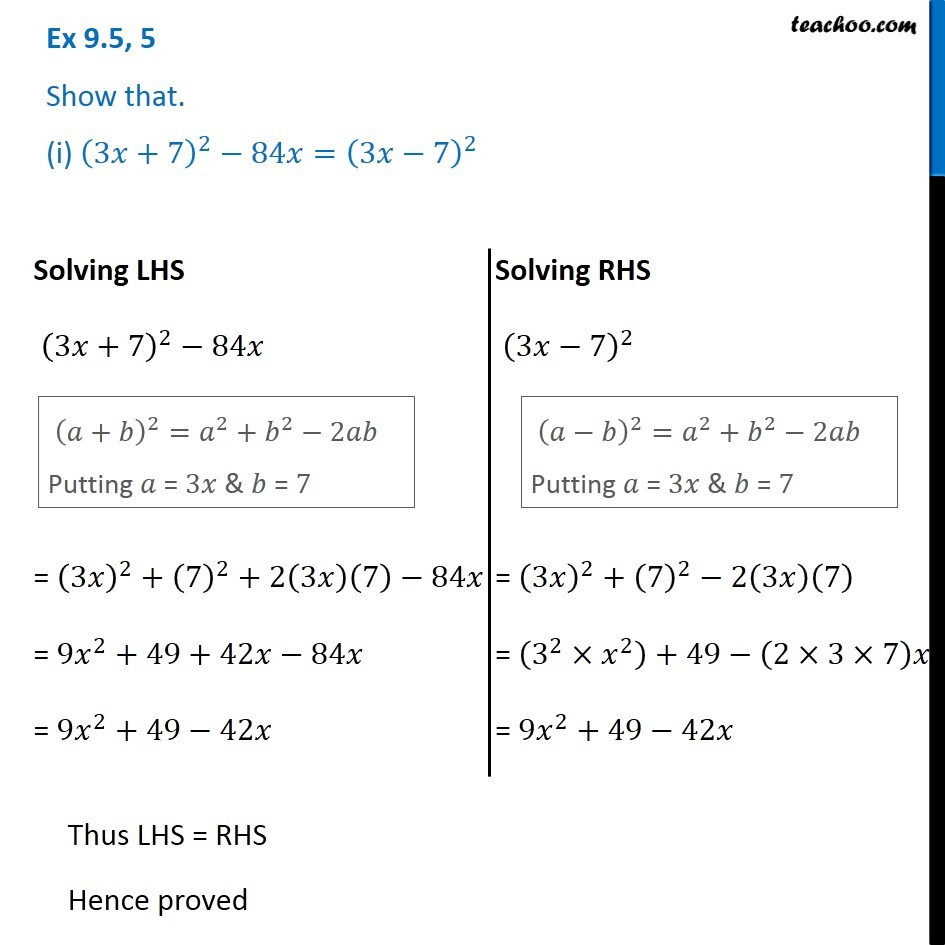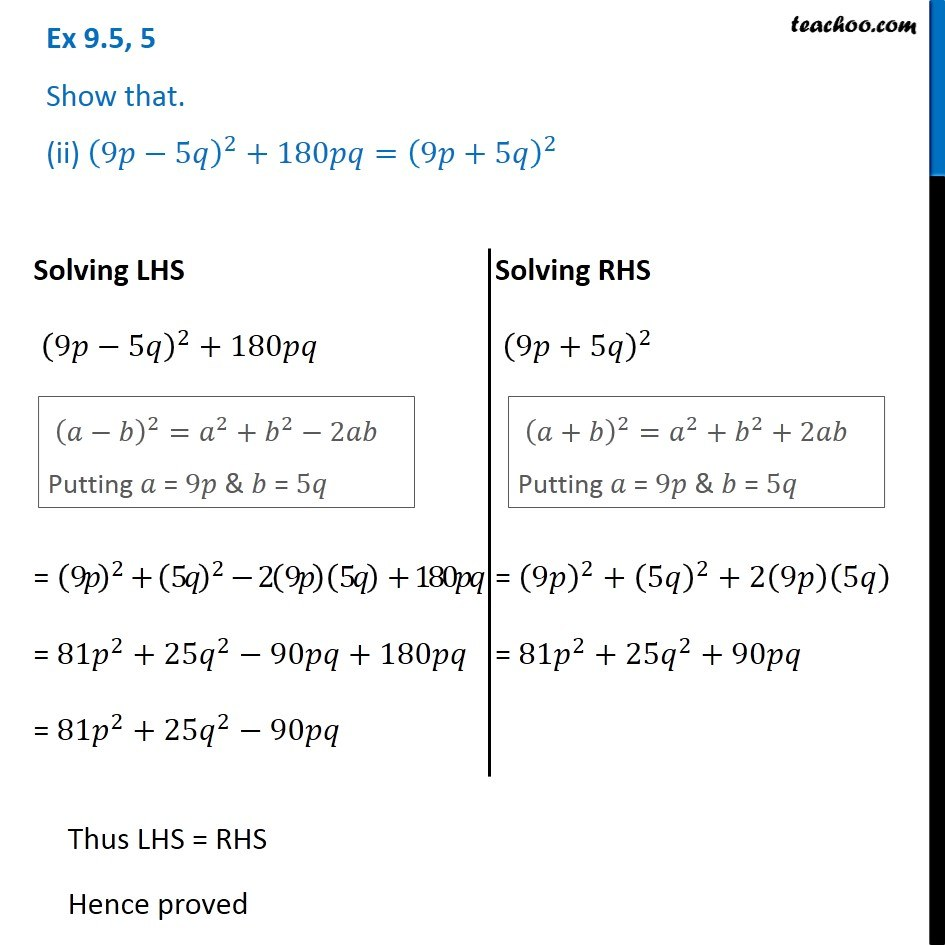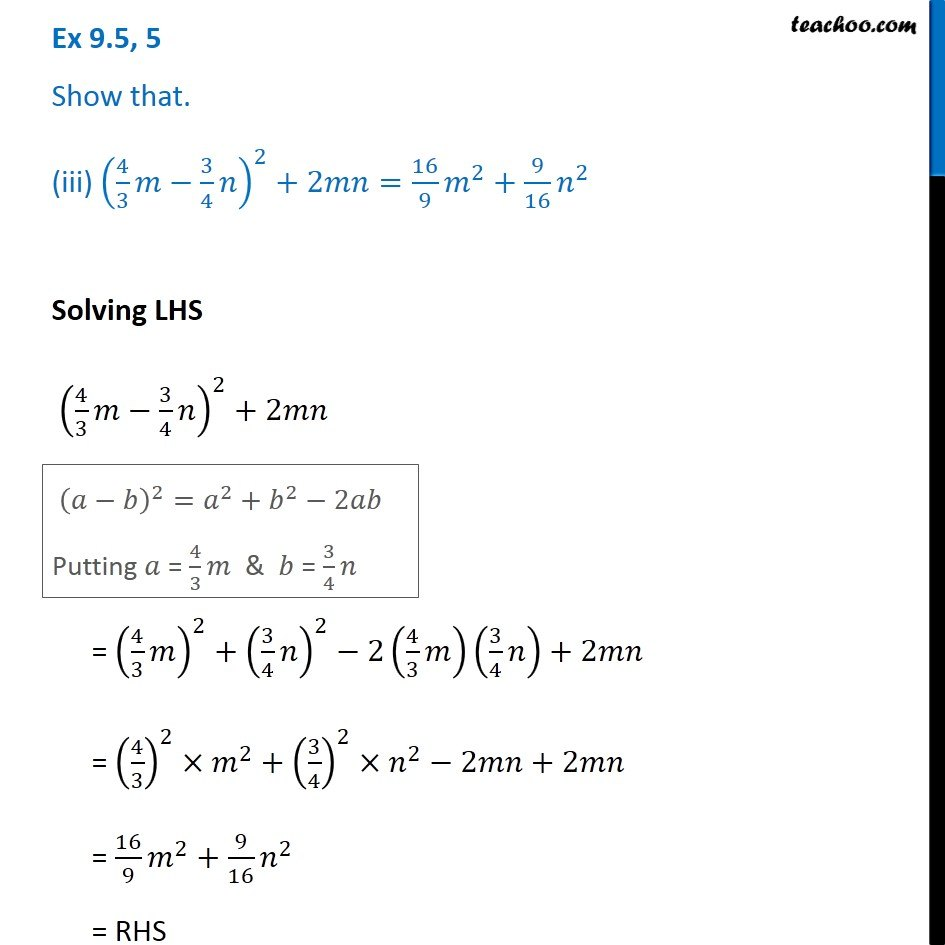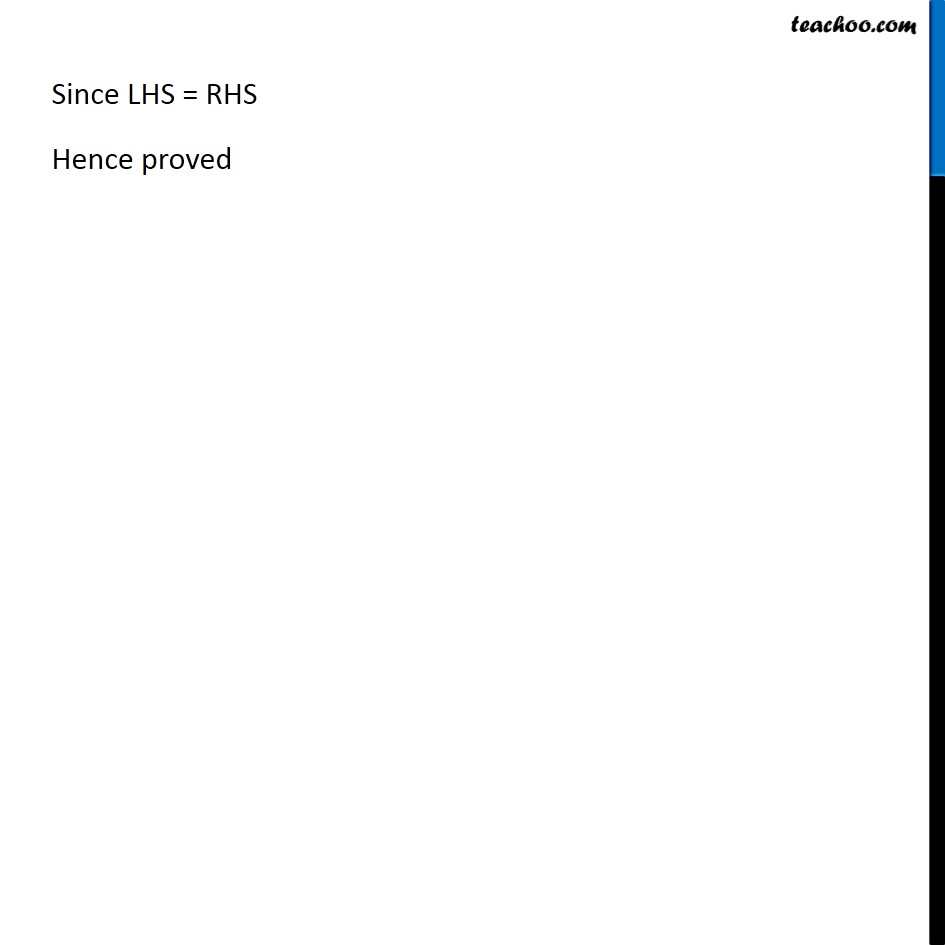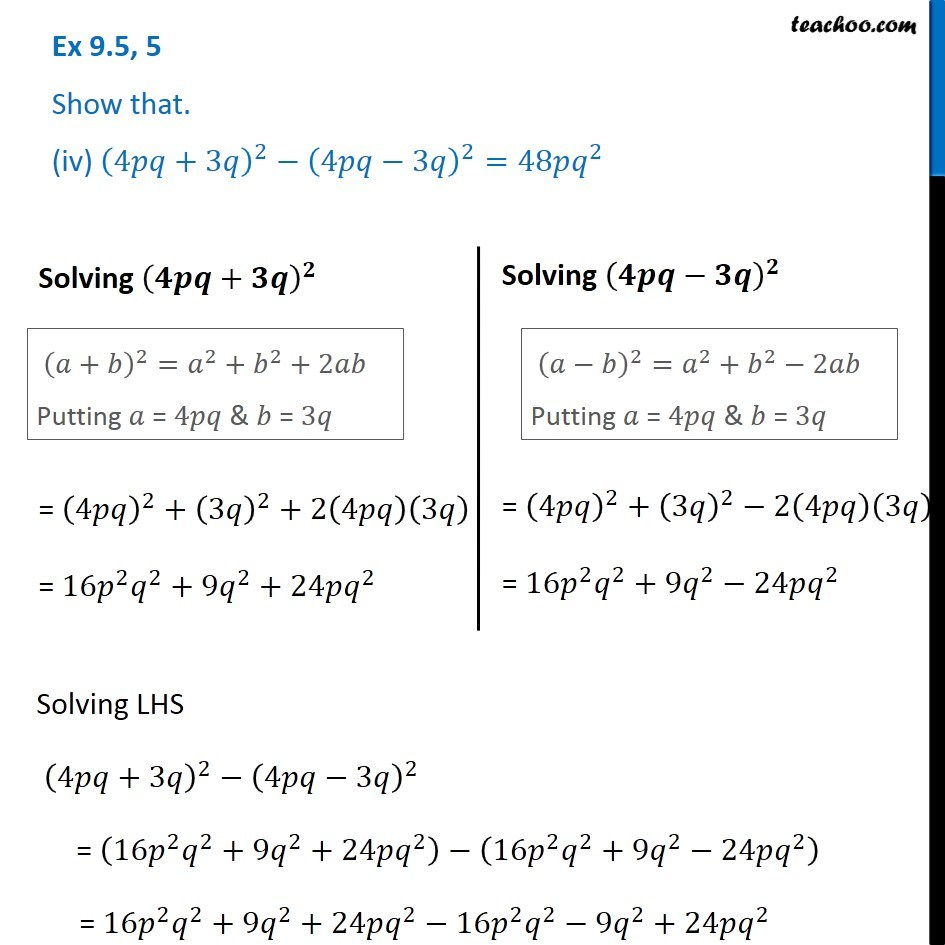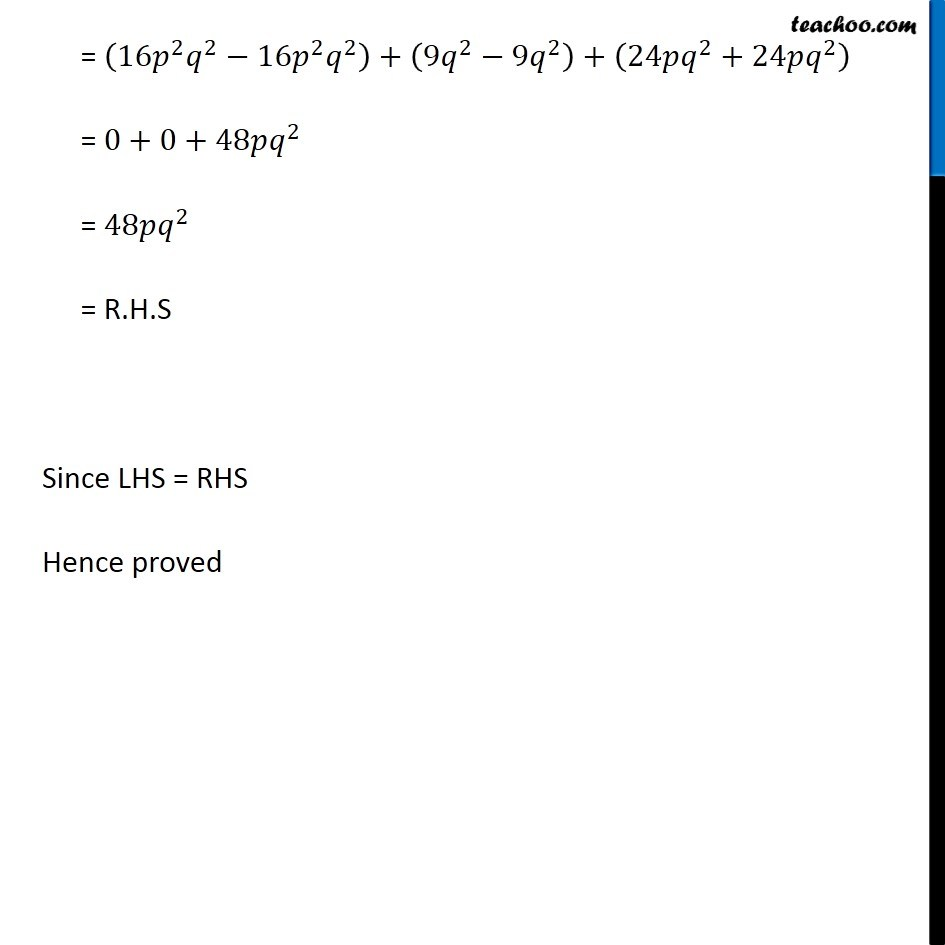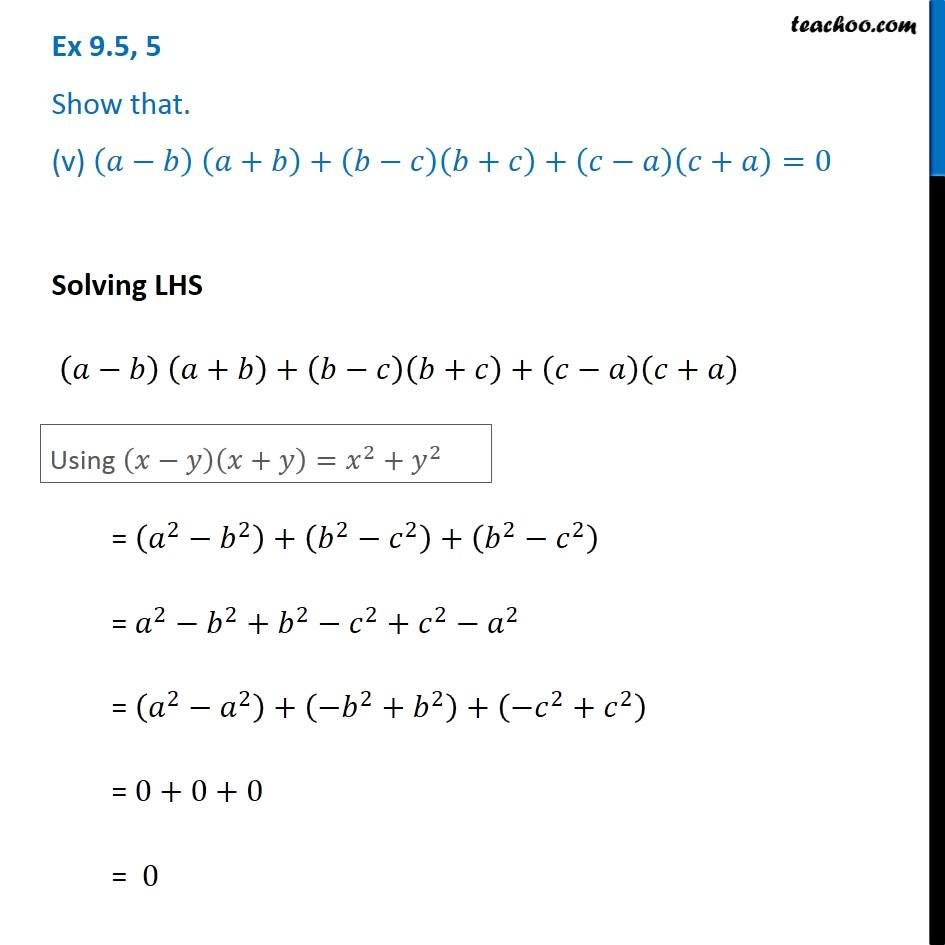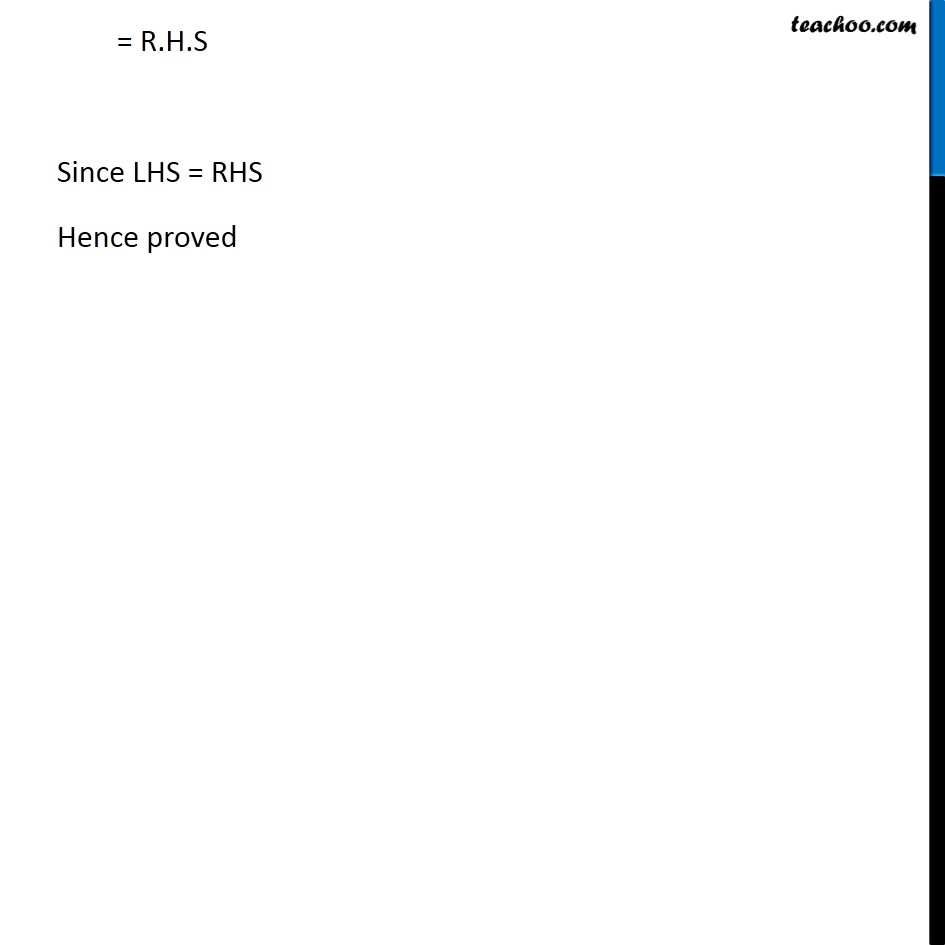1. Chapter 9 Class 8 Algebraic Expressions and Identities
2. Serial order wise
3. Ex 9.5

Transcript

Ex 9.5, 5 Show that. (i) (3𝑥+7)^2−84𝑥=(3𝑥−7)^2 Solving LHS (3𝑥+7)^2−84𝑥 = (3𝑥)^2+(7)^2+ 2(3𝑥)(7)−84𝑥 = 9𝑥^2+49+42𝑥−84𝑥 = 9𝑥^2+49−42𝑥 (𝑎+𝑏)^2=𝑎^2+𝑏^2−2𝑎𝑏 Putting 𝑎 = 3𝑥 & 𝑏 = 7 = (3𝑥)^2+(7)^2+ 2(3𝑥)(7)−84𝑥 = 9𝑥^2+49+42𝑥−84𝑥 = 9𝑥^2+49−42𝑥 Solving RHS (3𝑥−7)^2 (𝑎−𝑏)^2=𝑎^2+𝑏^2−2𝑎𝑏 Putting 𝑎 = 3𝑥 & 𝑏 = 7 = (3𝑥)^2+(7)^2−2(3𝑥)(7) = (3^2×𝑥^2 )+49−(2×3×7)𝑥 = 9𝑥^2+49−42𝑥 Thus LHS = RHS Hence proved Ex 9.5, 5 Show that. (ii) (9𝑝−5𝑞)^2+180𝑝𝑞=(9𝑝+5𝑞)^2 Solving LHS (9𝑝−5𝑞)^2+180𝑝𝑞 (𝑎−𝑏)^2=𝑎^2+𝑏^2−2𝑎𝑏 Putting 𝑎 = 9𝑝 & 𝑏 = 5𝑞 (𝑎−𝑏)^2=𝑎^2+𝑏^2−2𝑎𝑏 Putting 𝑎 = 9𝑝 & 𝑏 = 5𝑞 Solving RHS (9𝑝+5𝑞)^2 (𝑎+𝑏)^2=𝑎^2+𝑏^2+2𝑎𝑏 Putting 𝑎 = 9𝑝 & 𝑏 = 5𝑞 = (9𝑝)^2+(5𝑞)^2+2(9𝑝)(5𝑞) = 81𝑝^2+25𝑞^2+90𝑝𝑞 Thus LHS = RHS Hence proved Ex 9.5, 5 Show that. (iii) (4/3 𝑚−3/4 𝑛)^2+2𝑚𝑛=16/9 𝑚^2+9/16 𝑛^2 Solving LHS (4/3 𝑚−3/4 𝑛)^2+2𝑚𝑛 (𝑎−𝑏)^2=𝑎^2+𝑏^2−2𝑎𝑏 Putting 𝑎 = 4/3 𝑚 & 𝑏 = 3/4 𝑛 = (4/3 𝑚)^2+(3/4 𝑛)^2−2(4/3 𝑚)(3/4 𝑛)+2𝑚𝑛 = (4/3)^2×𝑚^2+(3/4)^2×𝑛^2−2𝑚𝑛+2𝑚𝑛 = 16/9 𝑚^2+9/16 𝑛^2 = RHS Since LHS = RHS Hence proved Ex 9.5, 5 Show that. (iv) (4𝑝𝑞+3𝑞)^2−(4𝑝𝑞−3𝑞)^2=48𝑝𝑞^2 Solving (𝟒𝒑𝒒+𝟑𝒒)^𝟐 (𝑎+𝑏)^2=𝑎^2+𝑏^2+2𝑎𝑏 Putting 𝑎 = 4𝑝𝑞 & 𝑏 = 3𝑞 (𝑎+𝑏)^2=𝑎^2+𝑏^2+2𝑎𝑏 Putting 𝑎 = 4𝑝𝑞 & 𝑏 = 3𝑞 = (4𝑝𝑞)^2+(3𝑞)^2+2(4𝑝𝑞)(3𝑞) = 16𝑝^2 𝑞^2+9𝑞^2+24𝑝𝑞^2 Solving (𝟒𝒑𝒒−𝟑𝒒)^𝟐 (𝑎−𝑏)^2=𝑎^2+𝑏^2−2𝑎𝑏 Putting 𝑎 = 4𝑝𝑞 & 𝑏 = 3𝑞 = (4𝑝𝑞)^2+(3𝑞)^2−2(4𝑝𝑞)(3𝑞) = 16𝑝^2 𝑞^2+9𝑞^2−24𝑝𝑞^2 Solving LHS (4𝑝𝑞+3𝑞)^2−(4𝑝𝑞−3𝑞)^2 = (16𝑝^2 𝑞^2+9𝑞^2+24𝑝𝑞^2 )−(16𝑝^2 𝑞^2+9𝑞^2−24𝑝𝑞^2 ) = 16𝑝^2 𝑞^2+9𝑞^2+24𝑝𝑞^2−16𝑝^2 𝑞^2−9𝑞^2+24𝑝𝑞^2 = (16𝑝^2 𝑞^2−16𝑝^2 𝑞^2 )+(9𝑞^2−9𝑞^2 )+(24𝑝𝑞^2+24𝑝𝑞^2 ) = 0+0+48𝑝𝑞^2 = 48𝑝𝑞^2 = R.H.S Since LHS = RHS Hence proved Ex 9.5, 5 Show that. (v) (𝑎−𝑏) (𝑎+𝑏)+(𝑏−𝑐)(𝑏+𝑐)+(𝑐−𝑎)(𝑐+𝑎)=0 Solving LHS (𝑎−𝑏) (𝑎+𝑏)+(𝑏−𝑐)(𝑏+𝑐)+(𝑐−𝑎)(𝑐+𝑎) Using (𝑥−𝑦)(𝑥+𝑦)=𝑥^2+𝑦^2 = (𝑎^2−𝑏^2 )+(𝑏^2−𝑐^2 )+(𝑏^2−𝑐^2 ) = 𝑎^2−𝑏^2+𝑏^2−𝑐^2+𝑐^2−𝑎^2 = (𝑎^2−𝑎^2 )+(−𝑏^2+𝑏^2 )+(−𝑐^2+𝑐^2 ) = 0+0+0 = 0 = R.H.S Since LHS = RHS Hence proved

Ex 9.5